# Continuation method (to a parametrized family, for non-linear operators)

A method for approximately solving non-linear operator equations. It consists of generalizing the equation to be solved,, to the form, by introducing a parameterthat takes values in a finite interval,, such that the initial equation is obtained for: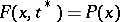, while the equationcan either easily be solved, or a solutionof it is already known (cf. ).

The generalized equation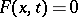is solved sequentially for individual values of: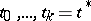. Forit is solved by means of some iteration method (Newton, simple iteration, variation of parameter, , etc.), starting with the solution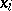obtained by solvingfor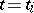. Applying at each step in, e.g.,Newton iterations, leads to the formulas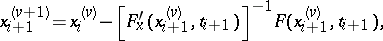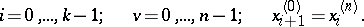If the difference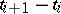is sufficiently small, then the value ofmay turn out to be a sufficiently good initial approximation, ensuring convergence, in order to obtain the solutionfor(cf. , , ).

In practice, the initial problem often naturally depends on some parameter, which can then be taken as.

The continuation method is used in the solution of systems of non-linear algebraic and transcendental equations (cf. , ), as well as for more general non-linear functional equations in Banach spaces (cf. ).

The continuation method is sometimes called the direct method of variation of parameter (cf. , ), as well as the combined method of direct and iterative variation of parameter. In these methods the construction of solutions of generalized equations is reduced, by differentiation with respect to the parameter, to the solution of a differential problem with initial conditions (a Cauchy problem) by methods of numerical integration of ordinary differential equations. Applying the simplest Euler method in the direct method of variation of parameter to the Cauchy problemthe approximate values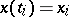,, of the solution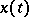ofcan be determined from the following identities: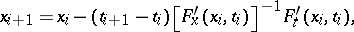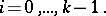The element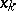is the required approximate solution of the initial equation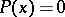. A refinement of all or some values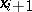can be obtained by the iteration method of variation of parameter  (or Newton's method). The generalized equation is here usually generated in the form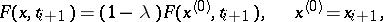on a finite interval, or, replacing in it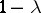by, on the infinite interval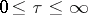.

The method of variation of parameter has been applied to large classes of problems both for constructing solutions, as well as for proving their existence (cf. e.g. , , , ).